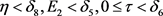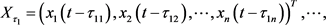# 一类经济大系统模型的建立及其Hopf分支Modeling a Large-Scale Economic System and Hopf Bifurcation

DOI: 10.12677/ORF.2019.91004, PDF, HTML, XML, 下载: 457  浏览: 964

Abstract: Taking the time delay non-linear large-scale system as the theoretical basis, the large-scale eco-nomic system is modeled, which takes the price of commodity as the key elements. Then, the sta-bility and Hopf bifurcation of the system model are studied by introducing a delay as a parameter. It is found that the Hopf bifurcation occurs when τ crosses some critical values. By using the center manifold method and normal form theory, some stability results are given for the periodic solution of the system.

1. 引言

2. 预备知识

$\left\{\begin{array}{l}{\stackrel{˙}{x}}_{1}\left(t\right)={c}_{11}\left(t\right){x}_{1}\left(t\right)+{c}_{12}\left(t\right){x}_{2}+{b}_{11}\left(t\right){x}_{1}\left(t-{\tau }_{11}\right)+{b}_{12}\left(t\right){x}_{2}\left(t-{\tau }_{12}\right)\\ {\stackrel{˙}{x}}_{2}\left(t\right)={c}_{21}\left(t\right){x}_{1}\left(t\right)+{c}_{22}\left(t\right){x}_{2}+{b}_{21}\left(t\right){x}_{1}\left(t-{\tau }_{21}\right)+{b}_{22}\left(t\right){x}_{2}\left(t-{\tau }_{22}\right)\end{array}$ (1)

$\left\{\begin{array}{l}{\stackrel{˙}{x}}_{1}\left(t\right)=-{a}_{11}\left(t\right){x}_{1}\left(t\right)+{a}_{12}\left(t\right){x}_{2}+{b}_{11}\left(t\right)\left[{x}_{1}\left(t-{\tau }_{11}\right)-{x}_{1}\left(t\right)\right]+{b}_{12}\left(t\right)\left[{x}_{2}\left(t-{\tau }_{12}\right)-{x}_{2}\left(t\right)\right]\\ {\stackrel{˙}{x}}_{2}\left(t\right)={a}_{21}\left(t\right){x}_{1}\left(t\right)-{a}_{22}\left(t\right){x}_{2}+{b}_{21}\left(t\right)\left[{x}_{1}\left(t-{\tau }_{21}\right)-{x}_{1}\left(t\right)\right]+{b}_{22}\left(t\right)\left[{x}_{2}\left(t-{\tau }_{22}\right)-{x}_{2}\left(t\right)\right]\end{array}$ (2)

${\stackrel{˙}{x}}_{1}\left(t\right)=-{a}_{11}\left(t\right){x}_{1}\left(t\right)+{a}_{12}\left(t\right){x}_{2},{\stackrel{˙}{x}}_{2}\left(t\right)={a}_{21}\left(t\right){x}_{1}\left(t\right)-{a}_{22}\left(t\right){x}_{2}$ (3)

${\stackrel{˙}{x}}_{1}\left(t\right)=-{a}_{11}\left(t\right){x}_{1},{\stackrel{˙}{x}}_{2}\left(t\right)=-{a}_{22}\left(t\right){x}_{2}$ (4)

$|{c}_{ij}\left(t\right)|\le \frac{a}{2},|{b}_{ij}\left(t\right)|\le \frac{a}{2}，|{a}_{ij}\left(t\right)|\le a\left(i,j=1,2,\forall t\ge {t}_{0}\right)$ (5)

$|{\stackrel{˙}{a}}_{ij}\left(t\right)|\le \epsilon \left(i,j=1,2,\forall t\ge {t}_{0}\right)$ (6)

${E}_{1}=\underset{{t}_{0}\le t\le +\infty }{\mathrm{max}}\left[|{a}_{12}\left(t\right)|,|{a}_{21}\left(t\right)|\right],\tau =\mathrm{max}\left({\tau }_{ij}\right)\left(i,j=1,2\right)$ (7)

${a}_{ii}\left(t\right)\ge {a}_{i}>0,\left(i=1,2,\forall t\ge {t}_{0}\right)$ (8)

${a}_{ii}\left(t\right)\le -{a}_{i}<0\left({a}_{i}>0,i=1,2,\forall t\ge {t}_{0}\right)$ (9)

${V}_{1}\left(t,{x}_{1}\right)=-{a}_{11}\left(t\right){x}_{1}^{2}>0,{V}_{2}\left(t,{x}_{2}\right)=-{a}_{22}\left(t\right){x}_{2}^{2}>0$ ，类似得到

${\stackrel{˙}{V}}_{i\left(4\right)}\ge \left(2{a}_{i}^{2}-\epsilon \right){x}_{i}^{2}>0,\left(i=1,2\right)$ (10)

${\stackrel{˙}{x}}_{i}\left(t\right)=\underset{j=1}{\overset{n}{\sum }}\left[{c}_{ij}\left(t\right){x}_{j}\left(t\right)+{b}_{ij}\left(t\right){x}_{j}\left(t-{\tau }_{ij}\right)\right],\left(i=1,2,\cdots ,n\right)$ (11)

${\stackrel{˙}{x}}_{i}\left(t\right)=\underset{j=1}{\overset{n}{\sum }}\left[{a}_{ij}\left(t\right){x}_{j}\left(t\right)+{b}_{ij}\left(t\right){x}_{j}\left(t-{\tau }_{ij}\right)-{x}_{j}\left(t\right)\right],\left(i=1,2,\cdots ,n\right)$ (12)

${\stackrel{˙}{x}}_{i}\left(t\right)=\underset{j=1}{\overset{n}{\sum }}{a}_{ij}\left(t\right){x}_{j}\left(t\right),\left(i=1,2,\cdots ,n\right)$ (13)

${\stackrel{˙}{X}}_{r}={A}_{{n}_{r}}\left(t\right){X}_{r},\left(r=1,\cdots ,m\right)$ (14)

$|{c}_{ij}\left(t\right)|\le \frac{a}{2},|{b}_{ij}\left(t\right)|\le \frac{a}{2},|{a}_{ij}\left(t\right)|\le a\left(i,j=1,2,\cdots ,n\right)\forall t\ge {t}_{0}$ (15)

$|{\stackrel{˙}{a}}_{ij}\left(t\right)|\le \epsilon \left(i,j={n}_{1}+\cdots {n}_{r-1}+1,\cdots ,{n}_{1}+\cdots +{n}_{r},r=1,\cdots ,m,{n}_{1}+\cdots +{n}_{m}=n,\forall t\ge {t}_{0}\right)$ (16)

$\tau =\mathrm{max}\left({\tau }_{ij}\right)\left(i,j=1,2,\cdots ,n\right)$ (17)

${E}_{2}=\underset{{t}_{0}\le t\le +\infty }{\mathrm{max}}\left[|{a}_{ij}\left(t\right)\right]$ (18)

$\left(\begin{array}{l}i=1,\cdots ,{n}_{1};j={n}_{1}+1,\cdots ,n;\\ i={n}_{1}+1,\cdots ,{n}_{1}+{n}_{2};j={n}_{1}+{n}_{2}+1,\cdots ,n;\\ i=n-{n}_{m}+1,\cdots ,n;j=1,\cdots ,n-{n}_{m}\end{array}\right)$

$\begin{array}{c}{D}_{r}\left(\lambda \left(t\right)\right)=\mathrm{det}|{A}_{{n}_{r}}\left(t\right)-\lambda I|\\ ={\lambda }^{{n}_{r}}\left(t\right)+{P}_{1}^{\left(r\right)}\left(t\right){\lambda }^{{n}_{r}-1}\left(t\right)+\cdots +{P}_{{n}_{r}-1}^{\left(r\right)}\left(t\right)\lambda \left(t\right)+{P}_{{n}_{r}}^{\left(r\right)}\left(t\right)\\ =0\left(r=1,\cdots ,m;{n}_{1}+\cdots +{n}_{m}=n,\forall t\ge {t}_{0}\right)\end{array}$ (19)

$\mathrm{Re}{\lambda }^{\left(r\right)}\left({A}_{{n}_{r}}\left(t\right)\right)\le -\delta <0\left(\delta >0,r=1,\cdots ,m,\forall t\ge {t}_{0}\right)$ (20)

$|{\stackrel{˙}{a}}_{ij}\left(t\right)|\le \epsilon <{\delta }_{4}\left(i,j={n}_{1}+\cdots +{n}_{r-1}+1,\cdots ,{n}_{1}+\cdots +{n}_{r};r=1,\cdots ,m,\forall t\ge {t}_{0}\right)$

$\tau =0$ 时，得到

$|{\stackrel{˙}{a}}_{ij}\left(t\right)|\le \epsilon <{\delta }_{4}\left(i,j={n}_{1}+\cdots +{n}_{r-1}+1,\cdots ,{n}_{1}+\cdots +{n}_{r};r=1,\cdots ,m,\forall t\ge {t}_{0}\right)$

$\begin{array}{c}{\stackrel{˙}{x}}_{i}\left(t\right)=\underset{j=1}{\overset{2}{\sum }}{c}_{ij}\left(t\right){x}_{j}\left(t\right)+\underset{j=1}{\overset{2}{\sum }}{b}_{ij}\left(t\right){x}_{j}\left(t-{\tau }_{ij}\right)+{f}_{i}\left(t,{x}_{1}\left(t\right),{x}_{2}\left(t\right),{x}_{1}\left(t-{\tau }_{i1}\right),{x}_{2}\left(t-{\tau }_{i2}\right)\right)\\ \left(i=1,2\right)\end{array}$ (21)

$\begin{array}{c}{\stackrel{˙}{x}}_{i}\left(\text{t}\right)=-{a}_{ii}\left(t\right){x}_{i}\left(t\right)+\underset{\begin{array}{c}j=1\\ j\ne i\end{array}}{\overset{2}{\sum }}{a}_{ij}\left(t\right){x}_{j}\left(t\right)\\ +\underset{j=1}{\overset{2}{\sum }}{b}_{ij}\left(t\right)\left[{x}_{j}\left(t-{\tau }_{ij}\right)-{x}_{j}\left(t\right)\right]+{f}_{i}\left(t,{x}_{1}\left(t\right),{x}_{2}\left(t\right),{x}_{1}\left(t-{\tau }_{i1}\right),{x}_{2}\left(t-{\tau }_{i2}\right)\right)\left(i=1,2\right)\end{array}$ (22)

$|{f}_{i}\left(t,{x}_{1},{x}_{2},{y}_{1},{y}_{2}\right)|\le \eta \left[\underset{i=1}{\overset{2}{\sum }}|{x}_{i}|+\underset{i=1}{\overset{2}{\sum }}|{y}_{i}|\right]\left(i=1,2\right)$ (23)

$|{\stackrel{˙}{a}}_{ij}\left(t\right)|\le \epsilon <{\delta }_{1}\left(i,j=1,2,\forall t\ge {t}_{0}\right){E}_{1}<{\delta }_{2},0\le \tau \le {\delta }_{3},\eta <{\delta }_{7}$

$\begin{array}{l}{\stackrel{˙}{x}}_{i}\left(t\right)=\underset{j=1}{\overset{n}{\sum }}\left[{c}_{ij}\left(t\right){x}_{j}\left(t\right)+{b}_{ij}\left(t\right){x}_{j}\left(t-{\tau }_{ij}\right)\right]+{f}_{i}\left(t,{x}_{1}\left(t\right),\cdots ,{x}_{n}\left(t\right),{x}_{1}\left(t-{\tau }_{i1}\right),{x}_{2}\left(t-{\tau }_{i2}\right),\cdots ,{x}_{n}\left(t-{\tau }_{in}\right)\right)\\ \left(i=1,\cdots ,n\right)\end{array}$ (24)

$\begin{array}{c}{\stackrel{˙}{x}}_{i}\left(t\right)=\underset{j=1}{\overset{n}{\sum }}\left[{a}_{ij}\left(t\right){x}_{j}\left(t\right)+{b}_{ij}\left(t\right)\left({x}_{j}\left(t-{\tau }_{ij}\right)-{x}_{j}\left(t\right)\right)\right]\\ +{f}_{i}\left(t,{x}_{1}\left(t\right),\cdots ,{x}_{n}\left(t\right),{x}_{1}\left(t-{\tau }_{i1}\right),{x}_{2}\left(t-{\tau }_{i2}\right),\cdots ,{x}_{n}\left(t-{\tau }_{in}\right)\right)\left(i=1,\cdots ,n\right)\end{array}$ (25)

$|{f}_{i}\left(t,{x}_{1},\cdots ,{x}_{n},{y}_{1},\cdots ,{y}_{n}\right)|\le \eta \left[\underset{i=1}{\overset{n}{\sum }}|{x}_{i}|+\underset{i=1}{\overset{n}{\sum }}|{y}_{i}|\right]\left(i=1,\cdots ,n\right)$ (26)

$|{\stackrel{˙}{a}}_{ij}\left(t\right)|\le \epsilon <{\delta }_{4}\left(i,j={n}_{1}+\cdots +{n}_{r-1}+1,\cdots ,{n}_{1}+\cdots +{n}_{r};r=1,\cdots ,m,\forall t\ge {t}_{0}\right)$$\begin{array}{c}{\stackrel{˙}{x}}_{i}\left(t\right)=\underset{j=1}{\overset{n}{\sum }}\left[{c}_{ij}{x}_{j}\left(t\right)+{b}_{ij}{x}_{j}\left(t-{\tau }_{ij}\right)\right]+{f}_{i}\left(t,{x}_{1}\left(t\right),\cdots ,{x}_{n}\left(t\right),{x}_{1}\left(t-{\tau }_{i1}\right),{x}_{2}\left(t-{\tau }_{i2}\right),\cdots ,{x}_{n}\left(t-{\tau }_{in}\right)\right)\\ \left(i=1,\cdots ,n\right)\end{array}$

${\stackrel{˙}{x}}_{i}\left(t\right)=\underset{j=1}{\overset{n}{\sum }}\left[{c}_{ij}{x}_{j}\left(t\right)+{b}_{ij}{x}_{j}\left(t\right)\right]+{f}_{i}\left(t,{x}_{1}\left(t\right),\cdots ,{x}_{n}\left(t\right)\right)\left(i=1,\cdots ,n\right)$

3. 主要结果

3.1. 经济大系统模型

$x={\left({x}_{1},{x}_{2},\cdots ,{x}_{n}\right)}^{T}\left(n\in {\mathcal{N}}^{+}\right)$ (27)

$A=\left[\begin{array}{cccc}{a}_{11}& {a}_{12}& \cdots & {a}_{1n}\\ {a}_{21}& {a}_{22}& \cdots & {a}_{2n}\\ ⋮& ⋮& \ddots & ⋮\\ {a}_{n1}& {a}_{n2}& \cdots & {a}_{nn}\end{array}\right]\left(n\in {\mathcal{N}}^{+}\right)$ (28)

${f}_{i}\left(t,{x}_{1},{x}_{2},\cdots ,{x}_{n}\right)\left(i=1,2,\cdots ,n,n\in {\mathcal{N}}^{+}\right)$ (29)

$x={\left({x}_{1}\left(t\right),{x}_{2}\left(t\right),\cdots ,{x}_{n}\left(t\right)\right)}^{T}\left(n\in {\mathcal{N}}^{+}，0\le t\le +\infty \right)$ (30)

$A=\left[\begin{array}{cccc}{a}_{11}\left(t\right)& {a}_{12}\left(t\right)& \cdots & {a}_{1n}\left(t\right)\\ {a}_{21}\left(t\right)& {a}_{22}\left(t\right)& \cdots & {a}_{2n}\left(t\right)\\ ⋮& ⋮& \ddots & ⋮\\ {a}_{n1}\left(t\right)& {a}_{n2}\left(t\right)& \cdots & {a}_{nn}\left(t\right)\end{array}\right]\left(n\in {\mathcal{N}}^{+}\right)$ (31)

${X}_{\tau }=\left[\begin{array}{cccc}{x}_{1}\left(t-{\tau }_{11}\right)& {x}_{2}\left(t-{\tau }_{12}\right)& \cdots & {x}_{2}\left(t-{\tau }_{12}\right)\\ {x}_{1}\left(t-{\tau }_{21}\right)& {x}_{2}\left(t-{\tau }_{22}\right)& \cdots & {x}_{2}\left(t-{\tau }_{n2}\right)\\ ⋮& ⋮& \ddots & ⋮\\ {x}_{1}\left(t-{\tau }_{n1}\right)& {x}_{2}\left(t-{\tau }_{n2}\right)& \cdots & {x}_{n}\left(t-{\tau }_{nn}\right)\end{array}\right]$ (32)

$\left(n\in {\mathcal{N}}^{+}\right),i,j=1,2,\cdots ,n,0\le {\tau }_{ij}

$\begin{array}{c}{f}_{i}\left(t,{x}_{1}\left(t\right),{x}_{2}\left(t\right),\cdots ,{x}_{n}\left(t\right),{x}_{1}\left(t-{\tau }_{i1}\right),{x}_{2}\left(t-{\tau }_{i2}\right),\cdots ,{x}_{n}\left(t-{\tau }_{in}\right)\right)\\ \left(i=1,2,\cdots ,n,n\in {\mathcal{N}}^{+}\right)\end{array}$ (33)(34)

$\begin{array}{c}\left[\begin{array}{c}{\stackrel{˙}{x}}_{1}\left(t\right)\\ {\stackrel{˙}{x}}_{2}\left(t\right)\\ ⋮\\ {\stackrel{˙}{x}}_{n}\left(t\right)\end{array}\right]=\left[\begin{array}{cccc}{a}_{11}\left(t\right)& {a}_{12}\left(t\right)& \cdots & {a}_{1n}\left(t\right)\\ {a}_{21}\left(t\right)& {a}_{22}\left(t\right)& \cdots & {a}_{2n}\left(t\right)\\ ⋮& ⋮& \ddots & ⋮\\ {a}_{n1}\left(t\right)& {a}_{n2}\left(t\right)& \cdots & {a}_{nn}\left(t\right)\end{array}\right]\left[\begin{array}{c}{x}_{1}\\ {x}_{2}\\ ⋮\\ {x}_{n}\end{array}\right]+\left[\begin{array}{c}{B}_{1}{X}_{{\tau }_{1}}\\ {B}_{2}{X}_{{\tau }_{2}}\\ ⋮\\ {B}_{n}{X}_{{\tau }_{n}}\end{array}\right]\\ +\left[\begin{array}{c}{f}_{1}\left(t,{x}_{1}\left(t\right),\cdots ,{x}_{n}\left(t\right),{x}_{1}\left(t-{\tau }_{11}\right),\cdots {x}_{n}\left(t-{\tau }_{1n}\right)\\ {f}_{2}\left(t,{x}_{1}\left(t\right),\cdots ,{x}_{n}\left(t\right),{x}_{1}\left(t-{\tau }_{21}\right),\cdots {x}_{n}\left(t-{\tau }_{2n}\right)\\ ⋮\\ {f}_{n}\left(t,{x}_{1}\left(t\right),\cdots ,{x}_{n}\left(t\right),{x}_{1}\left(t-{\tau }_{n1}\right),\cdots {x}_{n}\left(t-{\tau }_{nn}\right)\end{array}\right]\end{array}$ (35)

${B}_{1}=\left({b}_{11},{b}_{12},\cdots {b}_{1n}\right),\cdots ,{B}_{n}=\left({b}_{n1},{b}_{n2},\cdots ,{b}_{nn}\right)$ (36)$\begin{array}{c}{X}_{{\tau }_{n}}={\left({x}_{1}\left(t-{\tau }_{n1}\right),{x}_{2}\left(t-{\tau }_{n2}\right),\cdots ,{x}_{n}\left(t-{\tau }_{nn}\right)\right)}^{T}\\ \left(n\in {\mathcal{N}}^{+};i,j=1,2,\cdots ,n;0\le t\le +\infty ;0\le {\tau }_{ij} (37)

3.2. 模型解释

${\stackrel{˙}{x}}_{i}\left(t\right)$ 是商品价格随时间的变化，在此经济系统中，时间 $t$ 是唯一的参数，其他可能影响到商品价格的因素先忽略不计。

$Τ=\left[\begin{array}{cccc}t-{\tau }_{11}& t-{\tau }_{12}& \cdots & t-{\tau }_{1n}\\ t-{\tau }_{21}& t-{\tau }_{22}& \cdots & t-{\tau }_{2n}\\ ⋮& ⋮& \ddots & ⋮\\ t-{\tau }_{n1}& t-{\tau }_{n2}& \cdots & t-{\tau }_{nn}\end{array}\right]$ (38)

$\begin{array}{c}{\stackrel{˙}{x}}_{i}\left(t\right)=\underset{j=1}{\overset{n}{\sum }}\left[{a}_{ij}\left(t\right){x}_{j}\left(t\right)+{b}_{ij}\left(t\right){x}_{j}\left(t-{\tau }_{ij}\right)\right]+{f}_{i}\left(t,{x}_{1}\left(t\right),\cdots ,{x}_{n}\left(t\right),{x}_{1}\left(t-{\tau }_{i1}\right),{x}_{2}\left(t-{\tau }_{i2}\right),\cdots ,{x}_{n}\left(t-{\tau }_{in}\right)\right)\\ \left(i,j=1,2,\cdots ,n;0\le t\le +\infty ;0\le {\tau }_{ij} (39)

4. Hopf分支

$\begin{array}{c}P\left(\lambda ,{e}^{-\lambda {\tau }_{11}},{e}^{-\lambda {\tau }_{12}},\cdots ,{e}^{-\lambda {\tau }_{nn}}\right)={\lambda }^{{n}^{2}}+{p}_{1}^{0}{\lambda }^{{n}^{2}-1}+\cdots +{p}_{{n}^{2}}^{0}+\left({\lambda }^{{n}^{2}}+{p}_{1}^{1}{\lambda }^{{n}^{2}-1}+\cdots +{p}_{{n}^{2}}^{1}\right){e}^{-\lambda {\tau }_{11}}\\ +\cdots +\left({\lambda }^{{n}^{2}}+{p}_{1}^{{n}^{2}}{\lambda }^{{n}^{2}-1}+\cdots +{p}_{{n}^{2}}^{{n}^{2}}\right){e}^{-\lambda {\tau }_{nn}}\end{array}$ (40)

  刘永清, 宋中昆. 大型动力系统的理论与应用. 广州: 华南工学院出版社, 1988: 58-77.  马知恩, 周义仓, 李承治. 常微分方程定性与稳定性方法. 第二版. 北京: 科学出版社, 2015: 103-127.